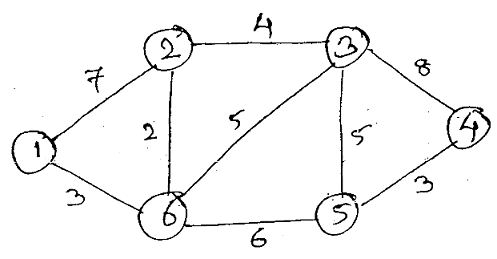MORE IN Analysis of Algorithms
MU Computer Engineering (Semester 4)
Analysis of Algorithms
May 2014
Total marks: --
Total time: --
INSTRUCTIONS
(1) Assume appropriate data and state your reasons
(2) Marks are given to the right of every question
(3) Draw neat diagrams wherever necessary

1(a) Explain 0, Ω and θ Notation with the help of graph.And represent the following function using above notations.
T(n)=3n+2
T(n) = 10n2-1
10 M
1(b) Explain 0/1 knapsack problem with example.
10 M

2(a) Write an algorithm of sum of subset.Solve following problem and draw portion of state space tree M =35,W=(5,7,10,12,15,18,20).
10 M
2(b) Explain longest common subsequence with example.
10 M

3(a) Explain all pair shortest path algorithm with suitable example.
10 M
3(b) Explain different string matching algorithms.
10 M

4(a) Write a Min Max function to find minimum and maximum value from given set of values using divide and conquer.Also drive its complexities.
10 M
4(b) Comment on any two modules of computation.
10 M

5(a) To find Dijkstra's shortest path from vertex 1 to vertex 4 for following graph.10 M
5(b) Explain flow shop scheduling with example.
10 M

Write notes on: (any two): -
6 (a) Job sequencing with deadlines
10 M
6 (b) Randomized Algorithm
10 M
6 (c) The 15 Puzzle problem.
10 M
6 (d) N-Queen problem.
10 M

More question papers from Analysis of Algorithms